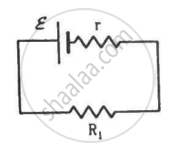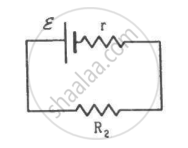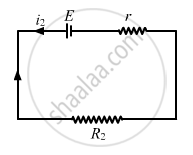Department of Pre-University Education, KarnatakaPUC Karnataka Science Class 12
Advertisement Remove all ads

# Two Unequal Resistances, R1 And R2, Are Connected Across Two Identical Batteries of Emf ε And Internal Resistance R (See the Figure). - Physics

Short Note

Two unequal resistances, R1 and R2, are connected across two identical batteries of emf ε and internal resistance r (see the figure). Can the thermal energies developed in R1 and R2 be equal in a given time? If yes, what will be the condition?Advertisement Remove all ads

#### SolutionFor the given time t, let the currents passing through the resistance R1 and R2 be i1 and i2, respectively.

Applying Kirchoff's Voltage Law to circuit-1, we get:-

$\epsilon - i_1 r - i_1 R_1 = 0$

$\Rightarrow i_1 = \frac{\epsilon}{r + R_1}$

Similarly, the current in the other circuit,

$i_2 = \frac{\epsilon}{r + R_2}$

The thermal energies through the resistances are given by

$i_1^2 R_1 t = i_2^2 R_2 t$

$\left( \frac{\epsilon}{r + R_1} \right)^2 R_1 t = \left( \frac{\epsilon}{r + R_2} \right)^2 R_2 t$

$\frac{R_1}{\left( r + R_1 \right)^2} = \frac{R_2}{\left( r + R_2 \right)^2}$

$\frac{\left( r^2 + {R_1}^2 + 2r R_1 \right)}{R_1} = \frac{\left( r^2 + {R_2}^2 + 2r R_2 \right)}{R_2}$

$\frac{r^2}{R_1} + R_1 = \frac{r^2}{R_2} + R_2$

$r^2 \left( \frac{1}{R_1} - \frac{1}{R_2} \right) = R_2 - R_1$

$r^2 \times \frac{R_2 - R_1}{R_1 R_2} = R_2 - R_1$

$r^2 = R_1 R_2$

$\Rightarrow r = \sqrt{R_1 R_2}$

Is there an error in this question or solution?
Advertisement Remove all ads

#### APPEARS IN

HC Verma Class 11, Class 12 Concepts of Physics Vol. 2
Chapter 11 Thermal and Chemical Effects of Current
Short Answers | Q 2 | Page 217
Advertisement Remove all ads

#### Video TutorialsVIEW ALL 

Advertisement Remove all ads
Share
Notifications

View all notifications

Forgot password?